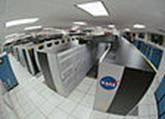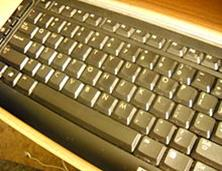# RAYS, ANGLES, TRIANGLES AND QUADRILATERALS. SOMETHING ABOUT EUCLIDEAN AND NON-EUCLIDEAN GEOMETRIESanother method of making sound, which is like vocal cords, or a voice box. Connected to the sound card are speakers, which are like a mouth, and are where the sound comes out. Computers might also have a graphics card, which helps the computer to create visual effects, such as 3D environments, or more realistic colours, and more powerful graphics cards can make more realistic or more advanced images, in the same way a well trained artist can.Drawing two rays originating from the same endpoint forms an angle Their common endpoint isthe vertex of the angle.

Angles, though open figures, separate the plane into 3 distinct sets of points: the interior, the exterior, the angle.

In the drawing above ray PA and ray PB form a right angle. Since ray PC (except for point P) lies in the interior of angle APB, we speak of angle CPA being less than aright angle and call it an acute angle. Since ray PD (except for point P) lies in the exterior ofangle APB, we say that angle APD is greater than a right angle and call it an obtuse angle. As far as triangles are concerned they may be classified according to the measures of the angles and the measures of the sides. Triangle ABC is referred to as an equilateral triangle. Triangle DEF is called an isosceles triangle which means that its two sides have the same measure. In triangle MKL, angle M is the right angle, sides MK and ML are called the legs, and side KL is called the hypotenuse. The hypotenuse refers only to the side opposite to the right angle of a right triangle. Quadrilaterals are geometric figures having four sides . A parallelogram is a quadrilateral whose opposite sides are parallel . A rectangle is a parallelogram in which all angles are right angles . A square is a rectangle having four congruent sides as well as

four right angles . A rhombus is a parallelogram in which the four sides are congruent. Thus it is evident that opposite sides of a rhombus are parallel and congruent.

CIRCLES.If you hold the sharp end of a compass fixed on a sheet of paper and then turn the compass completely around you will draw a curved line enclosing parts of a plane. It is a circle. A circle is a set of points in a plane each of which is equidistant, that is the same distance from some given point in the plane called the centre. A line segment joining any point of the circle with the centre is called a radius. A chord of a circle is a line segment. whose endpoints are points on the circle. A diameter is a chord which passes through the centre of the circle. Any part of a circle containing more than one point forms an arc of the circle. Instead of speaking of the perimeter of a circle, we usually use the term circumference to mean the distance around the circle. We cannot find the circumference of a circle by adding the measure of the segments, because a circle does not contain any segments.

No matter how short an arch is, it is curved at least slightly. Fortunately mathematicians have discovered that the ratio of the circumference (C) to a diameter (d) is the same for all circles. This ratio is expressed C / d. Since d = 2r (the length of a diameter is equal to twice the length of a radius of the same circle), the following denote the same ratio. C / d = C / 2r since d = 2r.

Дата добавления: 2015-12-01; просмотров: 1110; ЗАКАЗАТЬ НАПИСАНИЕ РАБОТЫ

Поиск по сайту:

При помощи поиска вы сможете найти нужную вам информацию, введите в поисковое поле ключевые слова и изучайте нужную вам информацию.

Поделитесь с друзьями:

Если вам понравился данный ресурс вы можете рассказать о нем друзьям. Сделать это можно через соц. кнопки выше.
helpiks.org - Хелпикс.Орг - 2014-2020 год. Материал сайта представляется для ознакомительного и учебного использования. | Поддержка
Генерация страницы за: 0.012 сек.